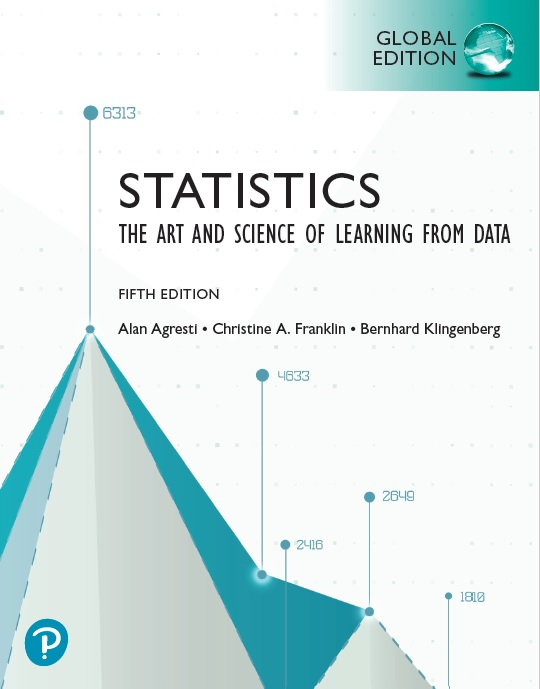# Statistics: The Art and Science of Learning from Data, 5th Edition PDF by Alan Agresti, Christine Franklin and Bernhard Klingenberg

## Statistics: The Art and Science of Learning from Data, Fifth Edition

By Alan Agresti, Christine Franklin and Bernhard KlingenbergContents:

Preface 10

Part One Gathering and Exploring Data

Chapter 1 Statistics: The Art and Science

of Learning From Data 24

1.1 Using Data to Answer Statistical Questions 25

1.2 Sample Versus Population 30

1.3 Organizing Data, Statistical Software, and the New Field of Data Science 42

Chapter Summary 52

Chapter Exercises 53

Chapter 2 Exploring Data With Graphs

and Numerical Summaries 57

2.1 Different Types of Data 58

2.2 Graphical Summaries of Data 64

2.3 Measuring the Center of Quantitative Data 82

2.4 Measuring the Variability of Quantitative Data 90

2.5 Using Measures of Position to Describe Variability 98

2.6 Linear Transformations and Standardizing 110

2.7 Recognizing and Avoiding Misuses of Graphical

Summaries 117

Chapter Summary 122

Chapter Exercises 125

Chapter 3 Exploring Relationships

Between Two Variables 134

3.1 The Association Between Two Categorical Variables 136

3.2 The Relationship Between Two Quantitative Variables 146

3.3 Linear Regression: Predicting the Outcome of a Variable 160

3.4 Cautions in Analyzing Associations 175

Chapter Summary 194

Chapter Exercises 196

Chapter 4 Gathering Data 204

4.1 Experimental and Observational Studies 205

4.2 Good and Poor Ways to Sample 213

4.3 Good and Poor Ways to Experiment 223

4.4 Other Ways to Conduct Experimental and

Nonexperimental Studies 228

Chapter Summary 240

Chapter Exercises 241

Part Two

Probability, Probability Distributions,

and Sampling Distributions

Chapter 5 Probability in Our Daily Lives 252

5.1 How Probability Quantifies Randomness 253

5.2 Finding Probabilities 261

5.3 Conditional Probability 275

5.4 Applying the Probability Rules 284

Chapter Summary 298

Chapter Exercises 300

Chapter 6 Random Variables and

Probability Distributions 307

6.1 Summarizing Possible Outcomes and Their

Probabilities 308

6.2 Probabilities for Bell-Shaped Distributions: The Normal

Distribution 321

6.3 Probabilities When Each Observation Has Two Possible

Outcomes: The Binomial Distribution 334

Chapter Summary 345

Chapter Exercises 347

Chapter 7 Sampling Distributions 354

7.1 How Sample Proportions Vary Around the Population Proportion 355

7.2 How Sample Means Vary Around the Population Mean 367

7.3 Using the Bootstrap to Find Sampling

Distributions 380

Chapter Summary 390

Chapter Exercises 392

Part Three Inferential Statistics

Chapter 8 Statistical Inference:

Confidence Intervals 400

8.1 Point and Interval Estimates of Population

Parameters 401

8.2 Confidence Interval for a Population Proportion 409

8.3 Confidence Interval for a Population Mean 426

8.4 Bootstrap Confidence Intervals 439

Chapter Summary 447

Chapter Exercises 449

Chapter 9 Statistical Inference:

9.1 Steps for Performing a Significance Test 458

9.2 Significance Test About a Proportion 464

9.3 Significance Test About a Mean 481

9.4 Decisions and Types of Errors in Significance Tests 493

9.5 Limitations of Significance Tests 498

9.6 The Likelihood of a Type II Error and the Power of a Test 506

Chapter Summary 513

Chapter Exercises 515

Chapter 10 Comparing Two Groups 522

10.1 Categorical Response: Comparing Two Proportions 524

10.2 Quantitative Response: Comparing Two Means 539

10.3 Comparing Two Groups With Bootstrap or Permutation Resampling 554

10.4 Analyzing Dependent Samples 568

10.5 Adjusting for the Effects of Other Variables 581

Chapter Summary 587

Chapter Exercises 590

Part Four

Extended Statistical Methods and Models for

Analyzing Categorical and Quantitative Variables

Chapter 11 Categorical Data Analysis 602

11.1 Independence and Dependence (Association) 603

11.2 Testing Categorical Variables for Independence 608

11.3 Determining the Strength of the Association 622

11.4 Using Residuals to Reveal the Pattern of Association 631

11.5 Fisher’s Exact and Permutation Tests 635

Chapter Summary 643

Chapter Exercises 645

Chapter 12 Regression Analysis 652

12.1 The Linear Regression Model 653

12.2 Inference About Model Parameters and the Relationship 663

12.3 Describing the Strength of the Relationship 670

12.4 How the Data Vary Around the Regression Line 681

12.5 Exponential Regression: A Model for Nonlinearity 693

Chapter Summary 698

Chapter Exercises 701

Chapter 13 Multiple Regression 707

13.1 Using Several Variables to Predict a Response 708

13.2 Extending the Correlation Coefficient and R2 for Multiple Regression 714

13.3 Inferences Using Multiple Regression 720

13.4 Checking a Regression Model Using Residual Plots 731

13.5 Regression and Categorical Predictors 737

13.6 Modeling a Categorical Response: Logistic Regression 743

Chapter Summary 752

Chapter Exercises 754

Chapter 14 Comparing Groups: Analysis of Variance Methods 760

14.1 One-Way ANOVA: Comparing Several Means 761

14.2 Estimating Differences in Groups for a Single Factor 772

14.3 Two-Way ANOVA: Exploring Two Factors and Their Interaction 781

Chapter Summary 795

Chapter Exercises 797

Chapter 15 Nonparametric Statistics 804

15.1 Compare Two Groups by Ranking 805

15.2 Nonparametric Methods for Several Groups and for

Dependent Samples 816

Chapter Summary 827

Chapter Exercises 829

Appendix A-833

Index I-865

Index of Applications I-872

Credits C-877

This book is US\$10
To get free sample pages OR Buy this book
Send email: [email protected]